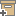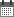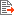ניהול
קהילה:
אסיף מאגר המחקר החקלאי
A geostatistical approach to the trickle irrigation design in heterogeneous soil: 1. TheoryBack to searchPrevious item
Next item
Year:
1983
Source of publication :
Water Resources Research
Authors :
רוסו, דוד
;
.
Volume :
19
Co-Authors:
Russo, D.
Facilitators :
From page:
632
To page:
642
(
Total pages:
11
)
Abstract:
A geostatistical approach was used to investigate the spatial variability of two measured soil hydraulic parameters, the saturated hydraulic conductivity Ks and α = d[log K(h)]/[d(h)], where K is the hydraulic conductivity and h is the soil water pressure head. Using a linearized solution to steady infiltration from a circular shallow pond, the spatial distribution of the midway soil water pressure head between emitters, hc (for a given trickle discharge rate Q and spacing between emitters, d), was calculated using the spatially variable soil parameters Ks and α. The spatial distribution of the crop yield Y was calculated from the spatially variable hc by using a quadratic expression for the Y(hc) relationships. It was found that the spatial variability of each of the measured input parameters (Ks and α) or the calculated output variables (hc and Y) is expressed through the variogram γ and can be characterized in terms of the coefficient of variation CV and the integral scale J. In the case of log Ks, for which the sill is reached at relatively small range, two different γ were tested. Results showed that the kriged values of log Ks as well as the resultant values of hc = hc(Ks α; d, Q) were relatively insensitive to the selected γ. The spatial variability of hc reduced the average (over the field) crop yield that would have been obtained when hc was uniform throughout the field. The spatially variable soil parameters Ks and α were used to calculate the spatial distribution of the spacing between emitters (d) required to achieve (for a given trickle discharge) a prespecified value of hc, uniform throughout the field. It was found that the use of a spatially variable d increased the field crop yield by 3% relative to the yield that was obtained when a single‐valued d uniform throughout the field was used. The spatial distribution of d was combined with hydraulic principles to derive the spatial distribution of lateral diameter and length for engineering design requirements. Copyright 1983 by the American Geophysical Union.
Note:
Related Files :
HYDRAULICS
irrigation
Mathematical models
SOILS - Moisture Determination
עוד תגיות
תוכן קשור
More details
DOI :
10.1029/WR019i003p00632
Article number:
Affiliations:
Database:
סקופוס
Publication Type:
מאמר
;
.
Language:
אנגלית
Editors' remarks:
ID:
19780
Last updated date:
02/03/2022 17:27
Creation date:
16/04/2018 23:31Scientific Publication
A geostatistical approach to the trickle irrigation design in heterogeneous soil: 1. Theory
19
Russo, D.
A geostatistical approach to the trickle irrigation design in heterogeneous soil: 1. Theory
A geostatistical approach was used to investigate the spatial variability of two measured soil hydraulic parameters, the saturated hydraulic conductivity Ks and α = d[log K(h)]/[d(h)], where K is the hydraulic conductivity and h is the soil water pressure head. Using a linearized solution to steady infiltration from a circular shallow pond, the spatial distribution of the midway soil water pressure head between emitters, hc (for a given trickle discharge rate Q and spacing between emitters, d), was calculated using the spatially variable soil parameters Ks and α. The spatial distribution of the crop yield Y was calculated from the spatially variable hc by using a quadratic expression for the Y(hc) relationships. It was found that the spatial variability of each of the measured input parameters (Ks and α) or the calculated output variables (hc and Y) is expressed through the variogram γ and can be characterized in terms of the coefficient of variation CV and the integral scale J. In the case of log Ks, for which the sill is reached at relatively small range, two different γ were tested. Results showed that the kriged values of log Ks as well as the resultant values of hc = hc(Ks α; d, Q) were relatively insensitive to the selected γ. The spatial variability of hc reduced the average (over the field) crop yield that would have been obtained when hc was uniform throughout the field. The spatially variable soil parameters Ks and α were used to calculate the spatial distribution of the spacing between emitters (d) required to achieve (for a given trickle discharge) a prespecified value of hc, uniform throughout the field. It was found that the use of a spatially variable d increased the field crop yield by 3% relative to the yield that was obtained when a single‐valued d uniform throughout the field was used. The spatial distribution of d was combined with hydraulic principles to derive the spatial distribution of lateral diameter and length for engineering design requirements. Copyright 1983 by the American Geophysical Union.
Scientific Publication
You may also be interested in